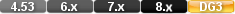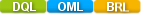Easy to Create, Easy to Change - Easy to use!## If Functionif( CONDITION, TRUE VALUE, FALSE VALUE) if(MyUser=current useer name, concat("This is correct", current user name),concat("This is wrong , current user name)) if(a=b,c,d)

The if function evaluates a specified condition and returns one of two specified values based on whether the condition is true or false.

In a field Derivation formula, you can use the if function to:

• derive a field value based on one of two specified values or expressions depending on whether a condition is true or false (see Example 1).

In a Validation formula, you can use the if function to:

• check a field's validity based on one of two specified expressions depending on whether a condition is true or false (see Example 2).

When you use an if function in a Derivation or Validation formula, the true value and false value parameters must be of the same data type as the field being derived or validated.

In a script, you can use the if function to:

• assign one of two specified values to a field or variable based on whether the condition is true or false (see Example3).

• hide or show a field, calculated value, or text string in a procedure's output depending on whether a condition is true or false (see Example 4).

A script, Derivation formula, or Validation formula may have multiple if function statements nested within one another.

# Parameters

CONDITION

Boolean condition i.e A>B A=B, A not = B etc.

TRUE VALUE

This is what happens if the condition is True. Can be any value, function or functions including further if functions.

FALSE VALUE

This is what happens if the condition is False. Can be any value, function or functions including further if functions.

# Returns/Result

The true value if the specified condition is true. The false value if the specified condition is false.

# Examples

Example 1

Using the if function in a Derivation formula:

The following example shows the iffunction used to derive the value in a Text field. The function returns one of two text values depending on the current time in the computer's system clock.

if( ampm( current time) = "AM" , " in the morning " ,  "in the afternoon" )

Because this Derivation formula returns a value for a Text field, the true value ("in the morning") and the false value ("in the afternoon") are both text expressions.

The following example shows the iffunction used in a Derivation formula for a Number field named DISCOUNT. The formula returns one of two DISCOUNT values depending on the value in another field, TOTAL DUE.

if( TOTAL DUE >= 500, TOTAL DUE * 0.15, TOTAL DUE * 0.03)

This formula evaluates the value in the TOTAL DUE field. If that value is greater than or equal to \$500 (making the condition true), the formula sets the value of DISCOUNT to TOTAL DUE * 0.15 If the value of TOTAL DUE is less than \$500 (making the condition false), the formula sets the value of DISCOUNT to TOTAL DUE * 0.03.

By nesting if statements inside one another, you can define a Derivation formula that evaluates multiple conditions and returns one of several values. The example below shows how one if statement can be nested inside another to return one of three DISCOUNT values depending on the value in the TOTAL DUE field:

if( TOTAL DUE >= 500, TOTAL DUE * 0.15,

if( TOTAL DUE >= 200, TOTAL DUE * 0.08, TOTAL DUE * 0.03) )

This formula evaluates the value in the TOTAL DUE field. If that value is greater than or equal to \$500 (making the condition true), the formula sets the value of DISCOUNT to the TOTAL DUE multiplied by 0.15. If the value is less than \$500 (making the condition false), a second if statement evaluates TOTAL DUE to determine if its value is greater than or equal to \$200. If this second condition is true, the value of DISCOUNT is set to the TOTAL DUE multiplied by 0.08. Otherwise DISCOUNT is set to TOTAL DUE * 0.03.

Example 2

Using the if function in a Validation formula:

The example below shows how the if function can be used in a Validation formula to conditionally apply one of two validation criteria to a field named CREDIT PURCHASES.

if( OVERDUE DAYS < 90, <=5000, <50)

This Validation formula evaluates the value in a field named OVERDUE DAYS (this field tracks a customer's past-due invoices). If that value is less than 90 (meaning the customer has no invoices over 90 days past due), then any value up to \$5000 is valid in the CREDIT PURCHASES field. If the value of OVERDUE DAYS exceeds 90, then only up to \$50 can be saved in the CREDIT PURCHASES field.

Example 3

Using the if function to assign a value in a DQL script:

The examples below show how to use the if function in a DQL script to conditionally assign a value to a field or variable depending on whether a condition is true or false.

modify records in RESERVATION AGENTS

BONUS := if( SALES > 80000, 1000, 0) .

This script assigns a value to the BONUS field in all RESERVATION AGENTS records. If an employee generated more than \$80,000 in sales last quarter, the value in the BONUS field is set to 1000. Otherwise the value of BONUS is set to zero.

The following line shows the if function used to assign a value to a temporary variable in a DQL script:

assign temp BONUS := if( SALES > 80000, 1000, 0) .

Example 4

You can use the if function to conditionally display fields, calculated values, or text strings in the output of a DQL procedure. The script below uses the if function to display the SALARY field in a procedure's printed output only if the procedure is run by a specific user:

for EMPLOYEES ;

list records

LAST NAME in order ;

FIRST NAME ;

JOB TITLE ;

if( current user name = "Moe" , SALARY, BLANK) .

end

This script tells DataEase: (1) For each record in the EMPLOYEES table, list the LAST NAME, FIRST NAME, and JOB TITLE, (2) evaluate the current user name system variable, and if the current user is Moe, list each employee's SALARY along with his/her other data, (3) otherwise, do not list the SALARY field.

# if Function

#### Type

Conditional Function

#### Purpose

The if function evaluates a specified condition and returns one of two specifiedvaluesbased on whether the condition is true or false.

Syntax

if(CONDITION, TRUE VALUE, FALSE VALUE)

Returns

The truevalueif the specified condition is true. The falsevalueif the specified condition is false.

Usage

In a field Derivation formula, you can use the if function to:

• derive a fieldvaluebased on one of two specifiedvaluesor expressions depending on whether a condition is true or false (see Example 1).

In a Validation formula, you can use the if function to:

• check a field's validity based on one of two specified expressions depending on whether a condition is true or false (see Example 2).

When you use an if function in a Derivation or Validation formula, the truevalueand falsevalueparameters must be of the same data type as the field being derived or validated.

In ascript, you can use the if function to:

• assignone of two specifiedvaluesto a field orvariablebased on whether the condition is true or false (see Example3).

• hide or show a field, calculatedvalue, or text string in a procedure'soutputdepending on whether a condition is true or false (see Example 4).

Ascript, Derivation formula, or Validation formula may have multiple if function statements nested within one another.

Example 1

Using the if function in a Derivation formula:

The following example shows the iffunction used to derive thevaluein a Text field. The function returns one of two textvaluesdepending on the current time in the computer's system clock.

if(ampm(current time)="AM","in the morning",

"in the afternoon")

Because this Derivation formula returns avaluefor a Text field, the truevalue("in the morning") and the falsevalue("in the afternoon") are both text expressions.

The following example shows the iffunction used in a Derivation formula for a Number field named DISCOUNT. The formula returns one of two DISCOUNTvaluesdepending on thevaluein another field, TOTAL DUE.

if(TOTAL DUE>=500, TOTAL DUE*0.15, TOTAL DUE*0.03)

This formula evaluates thevaluein the TOTAL DUE field. If thatvalueis greater than or equal to \$500 (making the condition true), the formula sets thevalueof DISCOUNT to TOTAL DUE * 0.15 If thevalueof TOTAL DUE is less than \$500 (making the condition false), the formula sets thevalueof DISCOUNT to TOTAL DUE * 0.03.

By nesting if statements inside one another, you candefinea Derivation formula that evaluates multiple conditions and returns one of severalvalues. The example below shows how one if statement can be nested inside another to return one of three DISCOUNTvaluesdepending on thevaluein the TOTAL DUE field:

if(TOTAL DUE>=500, TOTAL DUE*0.15,

if(TOTAL DUE>=200, TOTAL DUE*0.08, TOTAL DUE*0.03))

This formula evaluates thevaluein the TOTAL DUE field. If thatvalueis greater than or equal to \$500 (making the condition true), the formula sets thevalueof DISCOUNT to the TOTAL DUE multiplied by 0.15. If thevalueis less than \$500 (making the condition false), a second if statement evaluates TOTAL DUE to determine if itsvalueis greater than or equal to \$200. If this second condition is true, thevalueof DISCOUNT is set to the TOTAL DUE multiplied by 0.08. Otherwise DISCOUNT is set to TOTAL DUE * 0.03.

#### Example 2

Using the if function in a Validation formula:

The example below shows how the if function can be used in a Validation formula to conditionally apply one of two validation criteria to a field named CREDIT PURCHASES.

if(OVERDUE DAYS<90, <=5000, <50)

This Validation formula evaluates thevaluein a field named OVERDUE DAYS (this field tracks a customer's past-due invoices). If thatvalueis less than 90 (meaning the customer has no invoices over 90 days past due), then anyvalueup to \$5000 is valid in the CREDIT PURCHASES field. If thevalueof OVERDUE DAYS exceeds 90, then only up to \$50 can be saved in the CREDIT PURCHASES field.

Example 3

Using the if function toassignavaluein a DQLscript:

The examples below show how to use the if function in a DQLscriptto conditionallyassignavalueto a field orvariabledepending on whether a condition is true or false.

modify recordsinRESERVATION AGENTS

BONUS:=if(SALES>80000, 1000, 0).

Thisscriptassigns avalueto the BONUS field in all RESERVATION AGENTS records. If an employee generated more than \$80,000 in sales last quarter, thevaluein the BONUS field is set to 1000. Otherwise thevalueof BONUS is set to zero.

The following line shows the if function used toassignavalueto atemporaryvariablein a DQLscript:

assigntempBONUS:=if(SALES>80000, 1000, 0).

#### Example 4

You can use the if function to conditionally display fields, calculatedvalues, or text strings in theoutputof a DQL procedure. Thescriptbelow uses the if function to display the SALARY field in a procedure's printedoutputonly if the procedure is run by a specific user:

forEMPLOYEES;

list records

LAST NAMEin order;

FIRST NAME;

JOB TITLE;

if(current user name="Moe", SALARY, BLANK).

end

Thisscripttells DataEase: (1) For each record in the EMPLOYEES table, list the LAST NAME, FIRST NAME, and JOB TITLE, (2) evaluate the current user name systemvariable, and if the current user is Moe, list each employee's SALARY along with his/her other data, (3) otherwise, do not list the SALARY field.